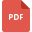# mathematics grade 4 united states tutorialsCounting with Negative Numbers
Mathematics, Grade 4, United States, Number SystemDefine and Sketch Triangles
Triangle, Grade 4, United States, MathematicsEquivalent Fraction and their Identification
Fraction, Grade 4, United States, MathematicsRectangles: Area and Perimeter Worksheet
Area, Grade 4, United States, Perimeter, Geometry, MathematicsComparing Decimals and their Arrangement
Mathematics, Grade 4, United States, FractionFraction, Grade 4, United States, MathematicsPractice Worksheet of Probability
Probability, Grade 4, United States, MathematicsMoney Word Problems, Grade 4, United States, MathematicsA Brief Description of Fractions
Fraction, Grade 4, United States, MathematicsShapes and Angles: Classification of angles
Lines and Angles, Grade 4, United States, MathematicsYr 4 Maths Revision Booklet
Basic Math, Grade 4, United States, MathematicsOrdering Numbers with up to 3 Decimal Places
Decimal and Fractions, Grade 4, United States, MathematicsGeometric Nets: For 3-D Shapes
Geometry, Grade 4, United States, MathematicsLine Segments in the Coordinate Plane
Coordinate Geometry, Grade 4, United States, MathematicsMean, Mode, Median, and Outliers
Mathematics, Grade 4, United States, StatisticsDecimals and Fraction Equivalent for Tenths
Decimal and Fractions, Grade 4, United States, MathematicsWorksheet on Fractions with Solutions
Fraction, Grade 4, United States, MathematicsRatio and Rates Word/Story Problems
Mathematics, Grade 4, United States, Ratio and ProportionMultiplication and Division Word/Story Problems
Multiplication, Grade 4, United States, Money Word Problems, Mathematics, DivisionWorksheet on Number Line
Mathematics, Grade 4, United States, Number SystemBasic Review: Components of a Fraction
Fraction, Grade 4, United States, MathematicsThe Coordinate Plane: Basics Explained
Coordinate Geometry, Grade 4, United States, Mathematics